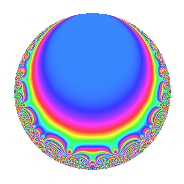# Properties

 Label 23.3.bLevel 23 Weight 3 Character orbit b Rep. character $$\chi_{23}(22,\cdot)$$ Character field $$\Q$$ Dimension 3 Newforms 1 Sturm bound 6 Trace bound 0

# Related objects

## Defining parameters

 Level: $$N$$ = $$23$$ Weight: $$k$$ = $$3$$ Character orbit: $$[\chi]$$ = 23.b (of order $$2$$ and degree $$1$$) Character conductor: $$\operatorname{cond}(\chi)$$ = $$23$$ Character field: $$\Q$$ Newforms: $$1$$ Sturm bound: $$6$$ Trace bound: $$0$$

## Dimensions

The following table gives the dimensions of various subspaces of $$M_{3}(23, [\chi])$$.

Total New Old
Modular forms 5 5 0
Cusp forms 3 3 0
Eisenstein series 2 2 0

## Trace form

 $$3q$$ $$\mathstrut +\mathstrut 12q^{4}$$ $$\mathstrut -\mathstrut 33q^{6}$$ $$\mathstrut -\mathstrut 21q^{8}$$ $$\mathstrut +\mathstrut 27q^{9}$$ $$\mathstrut +\mathstrut O(q^{10})$$ $$3q$$ $$\mathstrut +\mathstrut 12q^{4}$$ $$\mathstrut -\mathstrut 33q^{6}$$ $$\mathstrut -\mathstrut 21q^{8}$$ $$\mathstrut +\mathstrut 27q^{9}$$ $$\mathstrut +\mathstrut 3q^{12}$$ $$\mathstrut +\mathstrut 48q^{16}$$ $$\mathstrut +\mathstrut 39q^{18}$$ $$\mathstrut -\mathstrut 69q^{23}$$ $$\mathstrut -\mathstrut 132q^{24}$$ $$\mathstrut +\mathstrut 75q^{25}$$ $$\mathstrut +\mathstrut 87q^{26}$$ $$\mathstrut -\mathstrut 114q^{27}$$ $$\mathstrut -\mathstrut 84q^{32}$$ $$\mathstrut +\mathstrut 255q^{36}$$ $$\mathstrut -\mathstrut 42q^{39}$$ $$\mathstrut +\mathstrut 231q^{48}$$ $$\mathstrut +\mathstrut 147q^{49}$$ $$\mathstrut -\mathstrut 309q^{52}$$ $$\mathstrut -\mathstrut 297q^{54}$$ $$\mathstrut -\mathstrut 273q^{58}$$ $$\mathstrut +\mathstrut 78q^{59}$$ $$\mathstrut +\mathstrut 303q^{62}$$ $$\mathstrut -\mathstrut 45q^{64}$$ $$\mathstrut -\mathstrut 33q^{72}$$ $$\mathstrut +\mathstrut 399q^{78}$$ $$\mathstrut +\mathstrut 243q^{81}$$ $$\mathstrut -\mathstrut 129q^{82}$$ $$\mathstrut +\mathstrut 246q^{87}$$ $$\mathstrut -\mathstrut 276q^{92}$$ $$\mathstrut -\mathstrut 546q^{93}$$ $$\mathstrut -\mathstrut 57q^{94}$$ $$\mathstrut -\mathstrut 21q^{96}$$ $$\mathstrut +\mathstrut O(q^{100})$$

## Decomposition of $$S_{3}^{\mathrm{new}}(23, [\chi])$$ into irreducible Hecke orbits

Label Dim. $$A$$ Field CM Traces $q$-expansion
$$a_2$$ $$a_3$$ $$a_5$$ $$a_7$$
23.3.b.a $$3$$ $$0.627$$ 3.3.621.1 $$\Q(\sqrt{-23})$$ $$0$$ $$0$$ $$0$$ $$0$$ $$q+(\beta _{1}+\beta _{2})q^{2}+(-2\beta _{1}-\beta _{2})q^{3}+(4+\cdots)q^{4}+\cdots$$